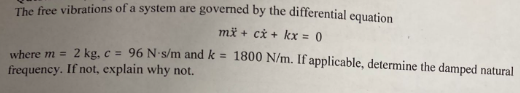Home / Expert Answers / Mechanical Engineering / the-free-vibrations-of-a-system-are-governed-by-the-differential-equation-mx-cx-kx-0-where-m-2-pa517

# (Solved): The free vibrations of a system are governed by the differential equation mx+cx+kx=0 where m=2 ...The free vibrations of a system are governed by the differential equation where and . If applicable, determine the damped natural frequency. If not, explain why not.

We have an Answer from Expert